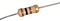# So what is Voltage, anyway?

## Voltage and Current explained in a simple, memorable way ✈

We use the term Voltage all the time. We know that AA batteries have 1.5 volts, a USB connector (or a phone charger) provides 5 volts, and when dealing with electronics, we usually work with either 5 volts, 3.3 volts, and sometimes higher numbers — car appliances operate on 12 volts, and mains is either 110 or 220 volts.

Despite the fact that Voltage is everywhere, I often see beginner questions where Voltage is confused with Current, Capacity or Energy. This is not surprising, considering that Voltage is often explained with vague terms such as “potential difference”, “electric field”, or “electric tension”, which only makes sense if you already come from a Physics background.

So what exactly is Voltage and how does is relate to Current? What does ground mean? Can you measure the voltage inside an electrical wire?

When giving beginner Electronics workshops, I always struggled when I got to the part where I explain about Voltage. I spent a lot of time thinking how I can explain it without relying on existing knowledge in electronics, when one day I came up with a simple analogy: Voltage is motivation. It’s how motivated electrons are to move from one point in the circuit to the other.

Amazingly, the first time I tried this explanation in a workshop, one of the student raised his hand and asked: “So, is it like when the cost of living in Tel-Aviv is higher than the one in Berlin, motivating people to relocate?”

At this moment, I knew it clicked. The more expensive Tel-Aviv is relative to Berlin, people are more motivated to move. Their level of motivation is the Voltage. Relocating, however, is not trivial — you have to pay for the flights. So if the flights are expensive, only the most motivated people will travel, but if the flights are cheap, the amount of people travelling will increase. The cost of flights is the equivalent of Electrical Resistance and the actual number of people moving from Tel-Aviv to Berlin every day resembles Current.

To reiterate, Voltage is how motivated are electrons to move from one point to another. Resistance is how hard is the way between these two points, and Current is how many electrons actually travel through a specific wire every second. The relation between these three is defined in a very simple formula, called Ohm’s law:

Voltage = Current × Resistance

This is the fundamental thing you need to know when you try to make sense of electronic circuits — in fact, many of the rules can be easily derived once you understand this fundamental relation.

When you have a power source (such as a battery), it has two leads: the positive and the negative. When these leads are disconnected from each other, there is no route for the electricity to flow, so the resistance is infinite. This means that there will be no current. Just like if there are no flights between Tel-Aviv and Berlin — even with the highest motivation, it won’t be possible to make this journey.

Similarly, if we short-circuit (touch the two leads together), there will be a nearly-zero resistance path between them, which means a lot of current will flow. This is similar to having many many airplanes or very large airplanes going between Tel-Aviv and Berlin, driving the air fare very low — even with the slightest motivation, you will still jump on a plane and make the trip.

When we connect a resistor between the leads, we can apply Ohm’s law to figure out the current. Let’s try it with some numbers — Assume we have a 1.5V battery, and 100Ω (Ohm) resistor. What would the current be? Using the above formula, we get:

1.5 = Current × 100

So the current equals `1.5 / 100`, or 0.015 Ampere (that’s 15mA). What happens when we connect another 100Ω resistor in parallel with the first one? We can use Ohm’s formula again, but let’s try to understand it intuitively instead. By adding a second resistor in parallel, we just added another path through which electrons can flow —it’s basically as if we added another daily flight between Tel Aviv and Berlin, or made our airplane twice as large. This means we can now drop prices. In other words, the resistance has decreased, so despite having the same level of motivation, more people can now travel, and the current will increase.

In contrast, adding a resistor in series is like stopping the direct flight service to Berlin so you now have to take a connection flight, for instance in London. This means the prices will go up — as now you have to pay for two flights instead of just one. So the resistance has increased, less people will be willing to pay for travelling, and the current will decrease.A standard 100Ω resistor: the colored bands correspond to the resistance value

In this post, I shared with you the mental model that I use when I think about voltage, current and resistance. I hope that this model will help you to understand these terms and the relation between them more intuitively and be useful for you when working on your next Arduino / electronics project.

If you want to learn more about the Physical behind electricity, check out this great tutorial from SparkFun. They also have another tutorial explaining Voltage/Current/Resistance in a more traditional way, using a water tank as an analogy.